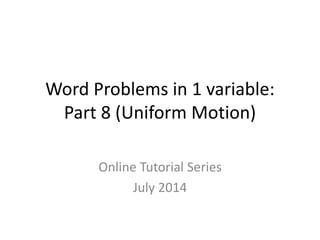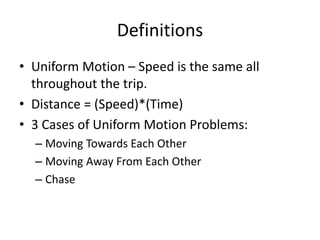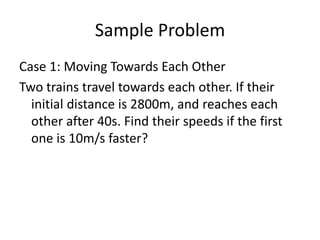Diese Präsentation wurde erfolgreich gemeldet.
Anzeige
Anzeige
Anzeige
Anzeige
Anzeige
Anzeige
Anzeige
Anzeige
Anzeige
Anzeige
Anzeige×

1 von 9 Anzeige

# Word8.pptx

Distance, speed, and time
Science
Science and technology
Two teucks, with the first one faster than the second by 20kph travel away from each other. Find their speed if after 15hrs they are 1,500km apart?

Distance, speed, and time
Science
Science and technology
Two teucks, with the first one faster than the second by 20kph travel away from each other. Find their speed if after 15hrs they are 1,500km apart?

Anzeige
Anzeige

### Word8.pptx

1. 1. Word Problems in 1 variable: Part 8 (Uniform Motion) Online Tutorial Series July 2014
2. 2. Definitions • Uniform Motion – Speed is the same all throughout the trip. • Distance = (Speed)*(Time) • 3 Cases of Uniform Motion Problems: – Moving Towards Each Other – Moving Away From Each Other – Chase
3. 3. Sample Problem Case 1: Moving Towards Each Other Two trains travel towards each other. If their initial distance is 2800m, and reaches each other after 40s. Find their speeds if the first one is 10m/s faster?
4. 4. Solution R: Let x be the speed of the slower train x+10 be the speed of the faster train E: time*speed = distance 40(x+10) + 40(x) = 2800 S: 40x + 400 + 40x = 2800 80x + 400 = 2800 80x = 2400; x=30, x+10=40 I: The first train’s speed is 40m/s, and 30/s for the second.
5. 5. Sample Problem Case 2: Moving Away From Each Other Two trucks, with the first one faster than the second by 20kph travels away from each other. Find their speeds if after 15 hours, they are 1500km apart?
6. 6. Solution R: Let x be the speed of the slower truck x+20 be the speed of the faster truck E: time*speed = distance 15(x+20) + 15(x) = 1500 S: 15x + 300 + 15x = 1500 30x + 300 = 1500 30x = 1200; x=40, x+20=60 I: The two trucks travel at speeds 40 and 60kph.
7. 7. Sample Problem Case 3: Chase A truck leave a toll gate at 5pm, with speed of 50kph. If a car leaves the same toll gate at 7pm, but with speed of 70kph, what time will the car meet the truck?
8. 8. Solution R: If the truck travelled at 5pm, then no matter how much in (t) hours the car travels, he travel (t+2) hours t -> time travelled by car t+2 -> time travelled by truck E: When they meet, they should have covered the same distance 50 (t+2) = 70 (t)
9. 9. Solution … 50 (t+2) = 70 (t) 50t + 100 = 70t 100 = 20t t=5hrs, or 5 hours after the car left or t+2=7hrs after the truck left I: They will meet at 12mn.